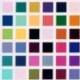# Statisfaction

## From SVG to probability distributions [with R package]

Posted in R, Statistics by Pierre Jacob on 25 August 2013

Hey,

To illustrate generally complex probability density functions on continuous spaces, researchers always use the same examples, for instance mixtures of Gaussian distributions or a banana shaped distribution defined on$\mathbb{R}^2$ with density function:$f(x,y) = \exp\left(-\frac{x^2}{200} - \frac{1}{2}(y+Bx^2-100B)^2\right)$

If we draw a sample from this distribution using MCMC we obtain a [scatter]plot like this one:Fig. 1: a sample from the very lame banana shaped distribution

Clearly it doesn’t really look like a banana, even if you use yellow to colour the dots like here. Actually it looks more like a boomerang, if anything. I was worried about this for a while, until I came up with a more realistic banana shaped distribution:Fig. 2: a sample from the realistic banana shaped distribution

See how the shape is well defined compared to the first figure? And there’s even the little tail, that proves so convenient when we want to peel off the fruit. More generally we might want to create target density functions based on general shapes. For this you can now try RShapeTarget, which you can install directly from R using devtools:

library(devtools)


The package parses SVG files representing shapes, and creates target densities from them. More precisely, a SVG files contains “paths”, which are sequence of points (for instance the above banana is a single closed path). The associated log density at any point$x$ is defined by$-1/(2\lambda) \times d(x, P)$ where$P$ is the closest path of the shape from$x$ and$d(x,P)$ is the distance between the point and the path. The parameter$\lambda$ specifies the rate at which the density decays when the point goes away from the shape. With this you can define the maple leaf distribution, as a tribute to JSM 2013:Fig. 3: a sample the “O Canada” probability distribution.

In the package you can get a distribution from a SVG file using the following code:

library(RShapeTarget)
# create target from file
my_shape_target <- create_target_from_shape(my_svg_file_name, lambda =1)
# test the log density function on 25 randomly generated points
my_shape_target$logd(matrix(rnorm(50), ncol = 2), my_shape_target$algo_parameters)


Since characters are just a bunch of paths, you can also define distributions based on words, for instance:Hodor: Hodor.

which is done as follows (warning you’re only allowed a-z and A-Z, no numbers no space no punctuation for now):

library(RShapeTarget)
word_target <- create_target_from_word("Hodor")


For the words, I defined the target density function as before, except that it’s constant on the letters: so if a point is outside a letter its density is computed based on the distance to the nearest path; if it’s inside a letter it’s just constant, so that the letters are “filled” with some constant density. I thought it’d look better.

Now I’m not worried about the banana shaped distribution any more, but by the fact that the only word I could think of was “Hodor” (with whom you can chat over there).

### 7 Responses

1.RINTU said, on 26 August 2013 at 06:20

nice!

2.xi'an said, on 27 September 2013 at 22:37

Hodor! Hodor…

3.Moustache target distribution and Wes Anderson | Statisfaction said, on 31 March 2014 at 16:51

[…] defines a target distribution represented by a SVG file using RShapeTarget. The target probability density function is defined on and is proportional to on the segments […]

4. […] defines a target distribution represented by a SVG file using RShapeTarget. The target probability density function is defined on and is proportional to on the segments […]

5.Moha said, on 28 May 2015 at 09:02

Hi,
when I use this command “word_target <- create_target_from_word("Hodor")"
I have this error: "Error: XML content does not seem to be XML: 'C:\Users\…\Documents\R\win-library\3.2\RShapeTarget\extdataH_uppercase_path.svg'

•Pierre Jacob said, on 28 May 2015 at 09:46